In this tutorial you will learn that how to remove leading or trailing spaces from a text string in a cell in Excel. This post will guide you how to delete leading or trailing spaces of all cells in a column.

If you remove leading spaces in cells one by one by manually, it will waste a lot of time. So this post will show you two methods to quickly remove leading and trailing spaces from text in one or more cells in your worksheet.

Assuming that you need to remove all leading spaces and trailing spaces in the range of cells A1:A4, you can refer to the following two methods to achieve it.

Method 1: Remove Leading and Trailing spaces with Excel Formula

To remove leading and trailing spaces from text string in Cells, you can use the TRIM function to remove extra spaces. So you can type the following formula in Cell B1, then press Enter key.

=TRIM(A1)

Then select Cell B1 and drag the AutoFill Handle down to other cells that you want to remove the leading or trailing spaces.

You will see that all of your leading and trailing spaces in range A1:A4 are cleaned up.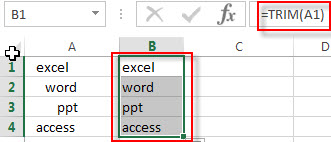Note: if the text contains non-braking spaces, this formula will not work, because the TRIM function is not able to remove non-breaking spaces. At this time, you should remove the non-breaking spaces firstly with the SUBSTITUTE function, and then use the TRIM function to remove the rest leading and trailing spaces. So you can write down the following Excel formula:

=TRIM(SUBSTITUTE(A1,CHAR(160)," "))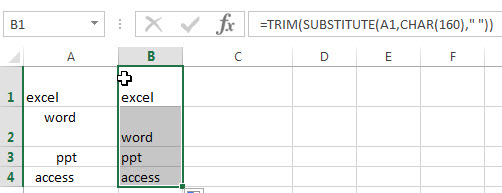You will see that all of leading and trailing spaces are removed from the text string in range A1:A4.

Or try to use the following formula:

=TRIM(CLEAN(SUBSTITUTE(A1,CHAR(160)," ")))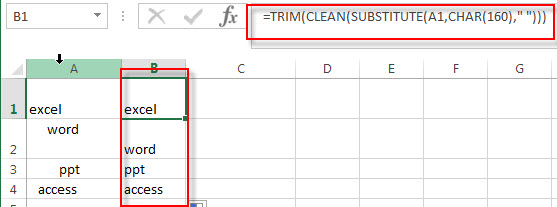The CHAR(160) formula returns a non-breaking space character.

The SUBSTITUTE function will replace all non-breaking space with new space character.

The CLEAN function will remove all non-printing characters, such as line breaks.

Method 2: Remove Leading and Trailing spaces with Excel VBA macro

If you want to quickly remove leading and trailing spaces, you can write an  Excel VBA macro code, just do it following:

1# click on “Visual Basic” command under DEVELOPER Tab.2# then the “Visual Basic Editor” window will appear.

3# click “Insert” ->”Module” to create a new module4# paste the below VBA code into the code window. Then clicking “Save” button.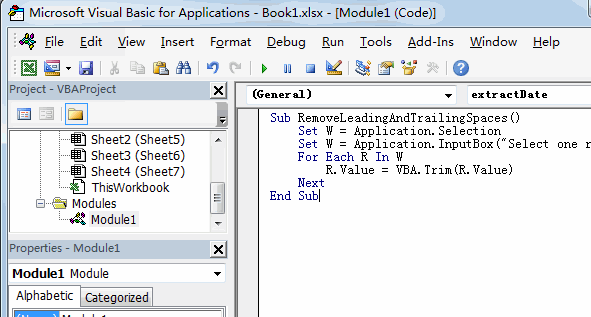Set W = Application.Selection
Set W = Application.InputBox("Select one range that you want to remove leading and trailing spaces:", "RemoveLeadingAndTrailingSpaces", W.Address, Type:=8)
For Each R In W
R.Value = VBA.Trim(R.Value)
Next
End Sub

5# back to the current worksheet, then run the above excel macro.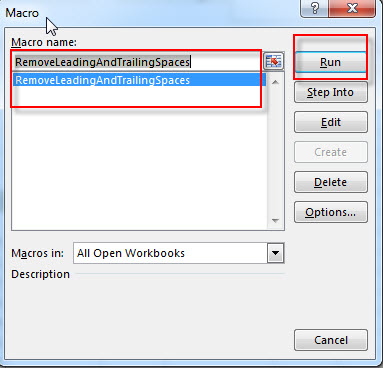6# select the range of cells that you want to remove leading and trailing spaces, then click OK button.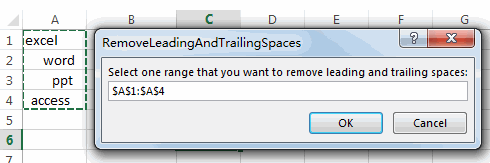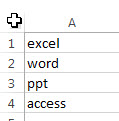Related Formulas

• count specific words in a cell or a range
If you want to count the number of a specific word in a single cell, you need to use the SUBSTITUTE function to remove all that certain word in text string, then using LEN function to calculate the length of the substring that without that specific word.…
• Extract word that starting with a specific character
Assuming that you have a text string that contains email address in Cell B1, and if you want to extract word that begins with a specific character “@” sign, you can use a combination with the TRIM function, the LEFT function, the SUBSTITUTE function, the MID function, the FIND function, the LEN function and the REPT function to create an excel formula.…
• Extract word that containing a specific character
If you want to extract word that contains a hash character in a text string in Cell B1, you can use a combination of the TRIM function, the MID function, the SUBSTITUTE function, the REPT function, the FIND function to create an excel formula.…
• Count the number of words in a cell
If you want to count the number of words in a single cell, you can create an excel formula based on the IF function, the LEN function, the TRIM function and the SUBSTITUTE function. ..
• Remove Spaces from a String
how to remove extra spaces between words or text string from the specified range of cells in excel. How to delete all spaces from excel cells. …

Related Functions

• Excel Substitute function
The Excel SUBSTITUTE function replaces a new text string for an old text string in a text string.The syntax of the SUBSTITUTE function is as below:= SUBSTITUTE  (text, old_text, new_text,[instance_num])….
• Excel CHAR function
The Excel CHAR function returns the character specified by a number (ASCII Value).The CHAR function is a build-in function in Microsoft Excel and it is categorized as a Text Function. The syntax of the CHAR function is as below:=CHAR(number)….
• Excel TRIM function
The Excel TRIM function removes all spaces from text string except for single spaces between words.  You can use the TRIM function to remove extra spaces between words in a string.The syntax of the TRIM function is as below:= TRIM (text)….
• Excel CLEAN function
The Excel CLEAN function removes all non-printable characters from a text string.The CLEAN function is a build-in function in Microsoft Excel and it is categorized as a Text Function.The syntax of the CLEAN function is as below:= CLEAN  (text)...

Related Posts

VBA Macro For VLOOKUP From Another Sheet

In the previous post, you should know that how to fix or remove the #N/A error when using VLOOKUP formula to lookup value from another sheet. And this post will show you how to use VBA code to vlookup data ...

How To Insert Comments in Protected Worksheet in Excel

This post will show you how to allow comments in a protected worksheet in Excel. You can easily to insert comments into cells in a normal worksheet in Excel, but if want to insert a comment in a worksheet that ...

How To Convert Text to Upper Cases(Using VBA) in Excel

This post will show you how to switch from lower case to upper case in Excel. and I am going to show you two different ways of converting text to upper cases using formula or VBA macro in Excel 2013,Excel ...

How To Hide Every Other Row in Excel (Using VBA)

This post will show you how to hide alternate rows or columns in Excel or how to hide every third, fourth, fifth row or column in Excel. If you want to hide every other row in your current worksheet, how ...

How to Disable the Save As Prompt in Excel

This post will show you how to use a VBA Macro to save an Excel file and overwrite any existing file without a prompt so that you are going to get the little window that says file already exists do ...

How to Count Cells that Contain even or odd numbers in Excel

This post will guide you how to count the number of cells that contain odd or even numbers within a range of cells using a formula in Excel 2013/2016.How do I count cells that contain odd numbers through the use ...

How to Count Cells that Contain negative Numbers in Excel

This post will guide you how to count the number of cells that contain negative numbers within a range of cells using a formula in Excel 2013/2016.You can count the number of negative numbers in your data using easy functions ...

How to Count Cells Are Not Blank or Empty in Excel

This post will guide you how to count cells that are not blank or empty in a given range cells using a formula in Excel 2013/2016.How do I count the number of cells that are not blank in a particular ...

How to Count Cells Not equal to X or Y in Excel

This post will guide you how to count the number of cells not equal to criteria X or Y in a given range cells using a formula in Excel 2013/2016.You can easily to count cells equal to or not equal ...

How to Count Cells Less Than a Specific Value in Excel

This post will guide you how to count the number of cells less than a particular numeric value in a given range cells using a formula in Excel 2013/2016. How do I count cells that are less than a specific ...

Sidebar